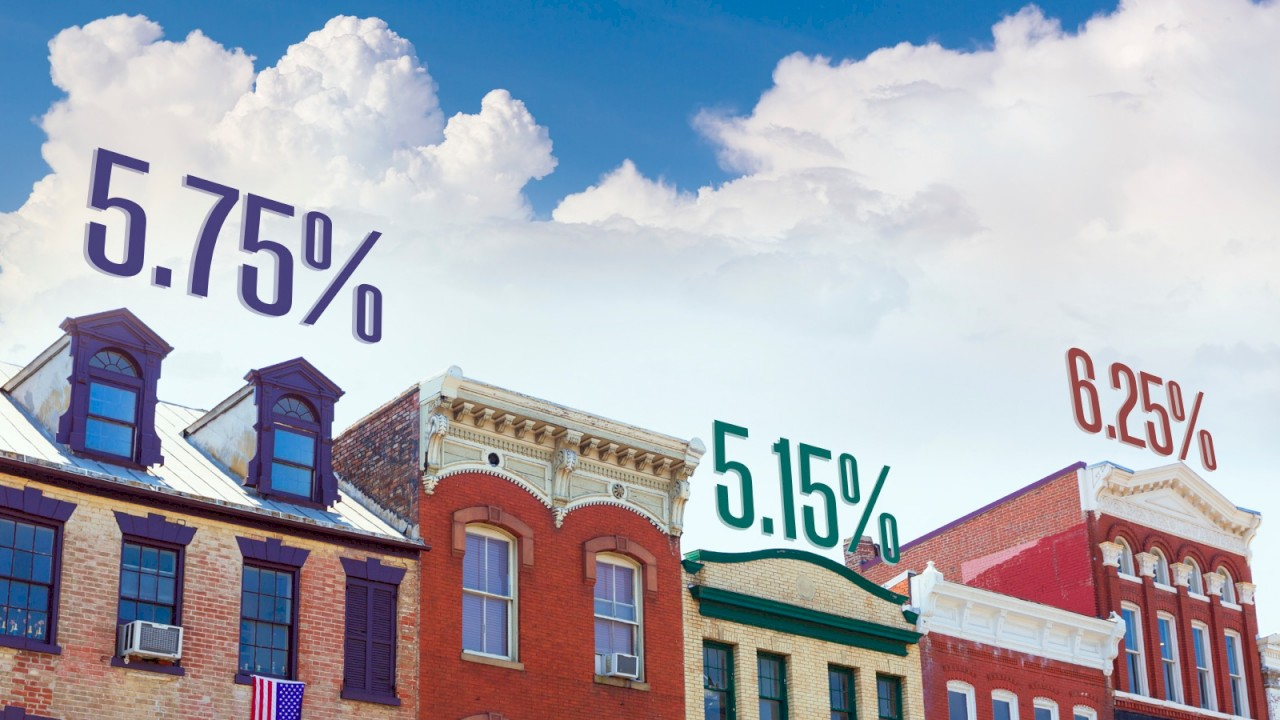What is Cap Rate
June 16, 2020Cap rates, or capitalization rates, are used by many agents, brokers, and investors as a quick way to determine the value of a property, or if a property is performing as desired. Finding the cap rate of a property is one of the first things I do when I’m looking at a property. It’s not the be all and end all to my property analysis, but it’s a good place to start when I’m looking at something listed for sale.

Unfortunately, it’s one of the most misunderstood principles used in real estate investing.

“The capitalization rate is the rate of return on a real estate investment property based on the income that the property is expected to generate. The capitalization rate is used to estimate the investor’s potential return on his or her investment” – Investopedia.com

In a nutshell, cap rate is the rate of return (cash) after expenses have been paid and before mortgage payments are made. The cap rate of a building is the same no matter the investor as it is based on the net operating income (NOI) and the value, or list price, of the property. What does change for each investor is the cap rate that he or she is happy with. This can be affected by many things, but one of the key factors of what cap rate an investor wants or needs is whether they are financing the property or not. It’s important to remember that the cap rate is the rate of return before any debt service payments are made.

## How to Calculate Cap Rate

While the principle and use of cap rates may be confusing, the math is actually very simple:

Cap Rate = NOI / Value

Cap rate is the net operating income divided by the value of the property

For example, an investor, Joan Smith, is considering purchasing a building that is listed for \$1,250,000. The seller has provided the income and expenses for the previous year, which give an NOI of \$80,000. Joan wants to know if this building has a good rate of return. We can determine the cap rate for the property as follows:

Cap Rate = 80,000 / 1,250,000

= 0.064 (6.4%)

This property has a 6.4% return based on the list price of \$1,250,000. If Joan does not need financing, then this is what she can expect for an annual rate of return with the income and expenses remaining as is. If she is financing the purchase, she may want a higher cap rate due to the fact that her mortgage payments have yet to be deducted.

## Using Cap Rate

The cap rate formula has three parts – cap rate, NOI and value. As long as you know two of those three factors, you can determine the third.

From the example above, a building is listed for \$1,250,000. Joan wants a cap rate of 8% or higher. In this case, we are calculating the minimum net income that the building needs to be producing:

NOI = value x cap rate

= 1,250,000 x 0.08

=\$100,000

Thus, the building should have a net income of at least \$100,000 (based on a price of \$1,250,000).

Finally, the value of a building can be determined if we know the cap rate and the NOI.

Value = NOI / cap rate

= 100,000 / 0.08

= \$1,250,000

Using this calculation, Joan can decide if the list price gives a good return on investment based on its income.